Probability as a measure of ignorance

One of the most beautiful intuitions about probability measures came from Rovelli’s book, that took it in turn from Bruno de Finetti.

What does a probability measure measure? Sure, the open sets of the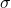-algebra that supports the measure space. But really, what? Thinking about it, it is very difficult to define probability without using the word probable or possible.

Well, probability measures our ignorance about something.

When we make some claim with 90% probability, what we are really saying is that the knowledge we have allows us to make a prediction that is that much accurate. And the main point here is that different people may assign different probabilities to the very same claim! If you have ever seen weather forecasts for the same day disagree, you know what I am talking about. Different data or different models can generate different knowledge, and thus different probability figures.

But we do not have to go that far to find reasonable examples. Let’s consider a very simple one. Imagine you found yourself on a train, and in front of you is sitting a girl with clothes branded Patagonia. What would be the odds that the girl has been to Patagonia? Not more than average, you would guess, because Patagonia is just a brand that makes warm clothes, and can be purchased in several stores all around the world, probably even more than in Patagonia itself! So you would probably say that is surely no more than 50% likely.

But now imagine a kid in the same scenario. If they see a girl with Patagonia clothes, they would immediately think that she had been to Patagonia (with probability 100% this time), because they are lacking a good amount of important information that you instead hold. And so the figure associated with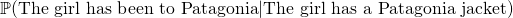is pretty different depending on the observer, or rather on the knowledge (or lack of) they possess. In this sense probability is a measure of our ignorance.

Conditional probability: why is it defined like that?

So, you want to calculate the probability of an event knowing that another has happened. There is a formula for that, it is called conditional probability, but why is it the way it is? Let’s first write down the definition of conditional probability: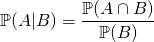We need to wonder: what does the happening of eventtell about the odds of happening of event? How much more likelybecomes ifhappens? Think in terms of howaffects.

Ifandare independent, then knowing something about B will not tell us anything at all about, at least not that we did not know already. In this case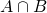is empty and thus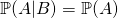. This makes sense! In fact, consider this example: how does me buying a copybook affects the likelihood that your grandma is going to buy a frying pan? It does not: the first event has no influence on the second, thus the conditional probability is just the same as the normal probability of the first event.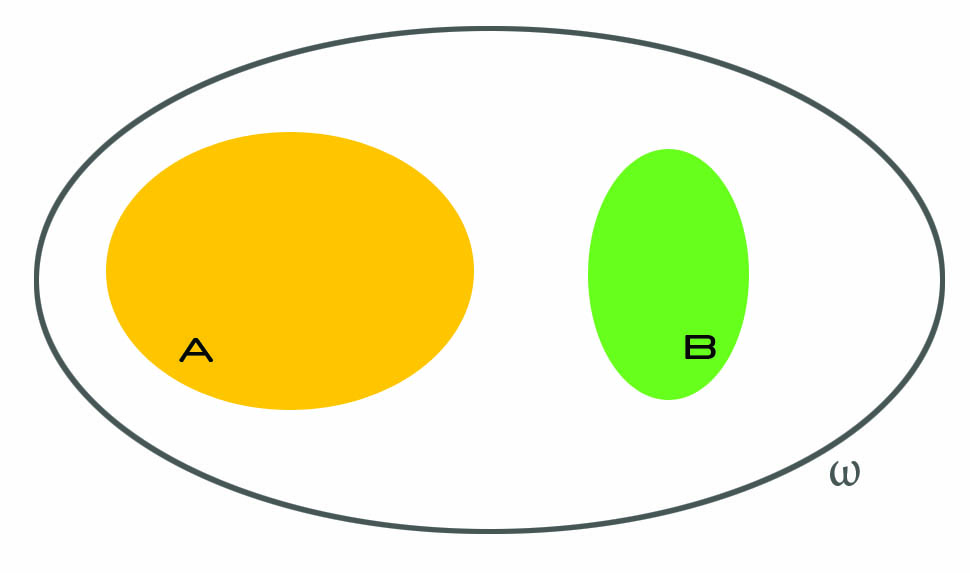Ifandare not independent, several things can happen, and that is where things get interesting. We know that B happened, and we should now think as ifwas our whole universe. The idea is: we already know what are the odds of, right? It is just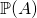. But how do they increase if we know that we do not really have to consider all possible events, but just a subset of them? As an example, think ofversusknowing that all balls are red. This makes a huge difference, right? (As an aside, that is what we mean when we say that probability is a measure of our ignorance.)

So anyway, now we ask: what is the probability of? Well, it would just be, but we must account for the fact that we now live inside, and everything that is outside it is as if it did not existed. Soactually becomes: we only care about the part ofthat is inside, because that is where we live now.

But, there is a caveat. Continue reading “Conditional probability: why is it defined like that?”

On the meaning of hypothesis and p-value in statistical hypothesis testing

Statistical hypothesis testing is really an interesting topic. I’ll just briefly sum up what statistical hypothesis testing is about, and what you do to test an hypothesis, but will assume you are already familiar with it, so that I can quickly cover a couple of A-HAs moments I had.

In statistical hypothesis testing, we

• have some data, whatever it is, which we imagine as being values of some random variable;
• make an hypothesis about the data, such as that the expected value of the random variable is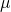;
• find a distribution for any affine transformation of the random variable we are making inference about – this is the test statistic;
• run the test, i.e. numerically say how much probable how observations were in relation to the hypothesis we made.

I had a couple of A-HA moments I’d like to share.

There is a reason why this is called hypothesis testing and not hypothesis choice. There are indeed two hypothesis, the null and the alternative hypothesis. However, their roles are widely different! 90% of what we do, both from a conceptual and a numerical point of view, has to do with the null hypothesis. They really are not symmetric. The question we are asking is “With the data I have, am I certain enough my null hypothesis no longer stands?” not at all “With the data I have, which of the two hypothesis is better?”

Metaphysics on geometric distribution in probability theory

I realized geometric distribution is not exactly about the time needed to get the first success in a given number of trials. This is a very odd feeling. It is probably a feeling applied mathematicians get sometimes, when they feel they are doing the best they can, and yet the theory is not perfect.

This may be a naive post, I warn you, but I was really stunned when I realized this.

Geometric distribution is not about the first success

Let’s jump to the point. We know (or at least, I was taught) that geometric distribution is used to calculate the probability that the first success in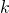trials (all independent and of probability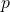) will happen precisely at the-th trial.

Remember that a geometric distribution is a random variable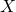such that its distribution is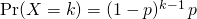How can we relate the above distribution with the fact that it matches the first success? Well, we need to have one success, which explains theat the bottom. Moreover, we want to have just one success, so all other trials must be unsuccessful, which explains the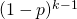.

But hey, where would first ever be written? Continue reading “Metaphysics on geometric distribution in probability theory”

Random variables: what are they and why are they needed?

This article aims at providing some intuition for what random variables are and why random variables are useful and needed in probability theory.

Intuition for random variables

Informally speaking, random variables encode questions about the world in a numerical way.

How many heads can I get if I flip a coin 3 times?

How many people will vote the Democrats at the US presidential elections?

I want to make pizza. What is the possible overall cost of the ingredients, considering all combinations of different brands of them?

These are all examples of random variables. What a random variable does, in plain words, is to take a set of possible world configurations and group them to a number. What I mean when I say world configurations will be clearer soon, when talking about the sample space(which, appropriately, is also called universe).